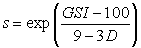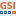# Generalized Hoek-Brown

The Generalized Hoek-Brown strength criterion is described by the following equation:where:is the uniaxial compressive strength (UCS) of the intact rock pieces

In most cases, it is practically impossible to carry out triaxial or shear tests on rock masses at a scale which is necessary to obtain direct values of the parameters in the Generalized Hoek-Brown equation. Therefore some practical means of estimating the material constants mb, s, and a is required. According to the latest research, the parameters of the Generalized Hoek-Brown criterion [Hoek, Carranza-Torres & Corkum (2002)], can be determined from the following equations:where:

• GSI is the Geological Strength Index
• mi is a material constant for the intact rock
• the parameter D is a "disturbance factor" which depends upon the degree of disturbance to which the rock mass has been subjected by blast damage and stress relaxation. It varies from 0 for undisturbed in situ rock masses to 1 for very disturbed rock masses.

The parameters GSI, mi and D can be estimated from these equations using the GSI Calculator dialog as described below.

## GSI Calculator

If you are using the Generalized Hoek-Brown criterion as the strength type for a material, the GSI Calculator dialog can provide valuable assistance to help you determine the parameters of the Generalized Hoek-Brown criterion for your material. To use the GSI Calculator dialog:

1. In the Material Properties dialog, set the Failure Criterion = Generalized Hoek-Brown.
2. Select the GSI buttonin the Material Properties dialog.
3. This will display the GSI Calculator dialog, which allows you to calculate the parameters mb, s, and a, from values of intact rock constant mi, GSI (Geological Strength Index), and Disturbance Factor, D, according to the equations of the Generalized Hoek-Brown criterion.
4. Enter values of GSI, mi, and D in the GSI Calculator dialog, and the corresponding values of mb, s, and a will automatically be displayed in the dialog.
5. You can use the Pick buttons to assist you in determining appropriate values of GSI, mi and D, see below for details.
6. Select OK to return to the Material Properties dialog, and the calculated values of mb, s, and a will automatically be loaded as the strength parameters for the material.

This is very useful because the parameters GSI, mi and D are usually the known quantities for a rock mass, whereas mb, s, and a are often not directly available.

### Pick GSI, mi, D, Intact UCS

Within the GSI Calculator dialog, you may access interactive tables and charts for:

• the estimation of mi based on rock type
• the estimation of GSI based on rock structure and surface conditions
• the estimation of Disturbance Factor based on excavation type and techniques
• the estimation of Intact Uniaxial Compressive Strength (UCS) based on field estimates

Select the Pick buttonfor the parameter that you wish to estimate, and you will see a table or chart which allows you to select an appropriate value for the parameter, for your material.

## Tensile Strength

See the Tensile Strength topic.